# 7 Types of Regression Techniques you should know!

## Introduction

Linear and Logistic regressions are usually the first algorithms people learn in predictive modeling. Due to their popularity, a lot of analysts even end up thinking that they are the only form of regressions. The ones who are slightly more involved think that they are the most important amongst all forms of regression analysis.

The truth is that there are innumerable forms of regressions, which can be performed. Each form has its own importance and a specific condition where they are best suited to apply. In this article, I have explained the most commonly used 7 forms of regressions in a simple manner. Through this article, I also hope that people develop an idea of the breadth of regressions, instead of just applying linear / logistic regression to every problem they come across and hoping that they would just fit

1. What is Regression Analysis?
2. Why do we use Regression Analysis?
3. What are the types of Regressions?
• Linear Regression
• Logistic Regression
• Polynomial Regression
• Stepwise Regression
• Ridge Regression
• Lasso Regression
• ElasticNet Regression
4. How to select the right Regression Model?

## What is Regression Analysis?

Regression analysis is a form of predictive modelling technique which investigates the relationship between a dependent (target) and independent variable (s) (predictor). This technique is used for forecasting, time series modelling and finding the causal effect relationship between the variables. For example, relationship between rash driving and number of road accidents by a driver is best studied through regression.

Regression analysis is an important tool for modelling and analyzing data. Here, we fit a curve / line to the data points, in such a manner that the differences between the distances of data points from the curve or line is minimized.  I’ll explain this in more details in coming sections.## Why do we use Regression Analysis?

As mentioned above, regression analysis estimates the relationship between two or more variables. Let’s understand this with an easy example:

Let’s say, you want to estimate growth in sales of a company based on current economic conditions. You have the recent company data which indicates that the growth in sales is around two and a half times the growth in the economy. Using this insight, we can predict future sales of the company based on current & past information.

There are multiple benefits of using regression analysis. They are as follows:

1. It indicates the significant relationships between dependent variable and independent variable.
2. It indicates the strength of impact of multiple independent variables on a dependent variable.

Regression analysis also allows us to compare the effects of variables measured on different scales, such as the effect of price changes and the number of promotional activities. These benefits help market researchers / data analysts / data scientists to eliminate and evaluate the best set of variables to be used for building predictive models.

## How many types of regression techniques do we have?

There are various kinds of regression techniques available to make predictions. These techniques are mostly driven by three metrics (number of independent variables, type of dependent variables and shape of regression line). We’ll discuss them in detail in the following sections.For the creative ones, you can even cook up new regressions, if you feel the need to use a combination of the parameters above, which people haven’t used before. But before you start that, let us understand the most commonly used regressions:

## 1. Linear Regression

It is one of the most widely known modeling technique. Linear regression is usually among the first few topics which people pick while learning predictive modeling. In this technique, the dependent variable is continuous, independent variable(s) can be continuous or discrete, and nature of regression line is linear.

Linear Regression establishes a relationship between dependent variable (Y) and one or more independent variables (X) using a best fit straight line (also known as regression line).

It is represented by an equation Y=a+b*X + e, where a is intercept, b is slope of the line and e is error term. This equation can be used to predict the value of target variable based on given predictor variable(s).The difference between simple linear regression and multiple linear regression is that, multiple linear regression has (>1) independent variables, whereas simple linear regression has only 1 independent variable.  Now, the question is “How do we obtain best fit line?”.

#### How to obtain best fit line (Value of a and b)?

This task can be easily accomplished by Least Square Method. It is the most common method used for fitting a regression line. It calculates the best-fit line for the observed data by minimizing the sum of the squares of the vertical deviations from each data point to the line. Because the deviations are first squared, when added, there is no cancelling out between positive and negative values.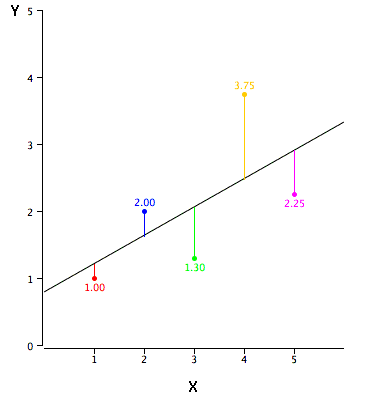We can evaluate the model performance using the metric R-square. To know more details about these metrics, you can read: Model Performance metrics Part 1, Part 2 .

#### Important Points:

• There must be linear relationship between independent and dependent variables
• Multiple regression suffers from multicollinearity, autocorrelation, heteroskedasticity.
• Linear Regression is very sensitive to Outliers. It can terribly affect the regression line and eventually the forecasted values.
• Multicollinearity can increase the variance of the coefficient estimates and make the estimates very sensitive to minor changes in the model. The result is that the coefficient estimates are unstable
• In case of multiple independent variables, we can go with forward selection, backward eliminationand step wise approach for selection of most significant independent variables.

## 2. Logistic Regression

Logistic regression is used to find the probability of event=Success and event=Failure. We should use logistic regression when the dependent variable is binary (0/ 1, True/ False, Yes/ No) in nature. Here the value of Y ranges from 0 to 1 and it can represented by following equation.

odds= p/ (1-p) = probability of event occurrence / probability of not event occurrence
ln(odds) = ln(p/(1-p))
logit(p) = ln(p/(1-p)) = b0+b1X1+b2X2+b3X3....+bkXk

Above, p is the probability of presence of the characteristic of interest. A question that you should ask here is “why have we used log in the equation?”.

Since we are working here with a binomial distribution (dependent variable), we need to choose a link function which is best suited for this distribution. And, it is logit function. In the equation above, the parameters are chosen to maximize the likelihood of observing the sample values rather than minimizing the sum of squared errors (like in ordinary regression).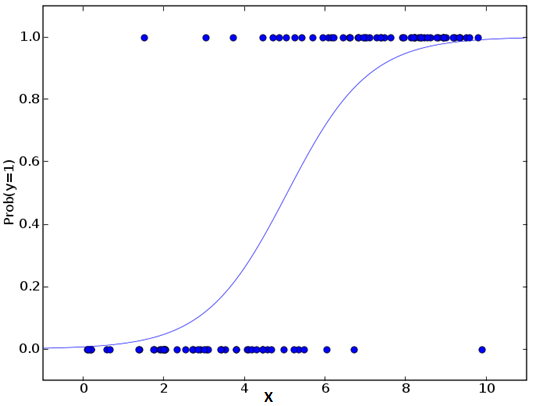#### Important Points:

• It is widely used for classification problems
• Logistic regression doesn’t require linear relationship between dependent and independent variables.  It can handle various types of relationships because it applies a non-linear log transformation to the predicted odds ratio
• To avoid over fitting and under fitting, we should include all significant variables. A good approach to ensure this practice is to use a step wise method to estimate the logistic regression
• It requires large sample sizes because maximum likelihood estimates are less powerful at low sample sizes than ordinary least square
• The independent variables should not be correlated with each other i.e. no multi collinearity.  However, we have the options to include interaction effects of categorical variables in the analysis and in the model.
• If the values of dependent variable is ordinal, then it is called as Ordinal logistic regression
• If dependent variable is multi class then it is known as Multinomial Logistic regression.

## 3. Polynomial Regression

A regression equation is a polynomial regression equation if the power of independent variable is more than 1. The equation below represents a polynomial equation:

y=a+b*x^2

In this regression technique, the best fit line is not a straight line. It is rather a curve that fits into the data points.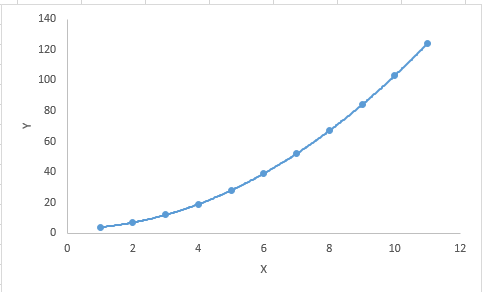#### Important Points:

• While there might be a temptation to fit a higher degree polynomial to get lower error, this can result in over-fitting. Always plot the relationships to see the fit and focus on making sure that the curve fits the nature of the problem. Here is an example of how plotting can help: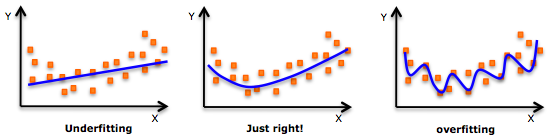• Especially look out for curve towards the ends and see whether those shapes and trends make sense. Higher polynomials can end up producing wierd results on extrapolation.

## 4. Stepwise Regression

This form of regression is used when we deal with multiple independent variables. In this technique, the selection of independent variables is done with the help of an automatic process, which involves no human intervention.

This feat is achieved by observing statistical values like R-square, t-stats and AIC metric to discern significant variables. Stepwise regression basically fits the regression model by adding/dropping co-variates one at a time based on a specified criterion. Some of the most commonly used Stepwise regression methods are listed below:

• Standard stepwise regression does two things. It adds and removes predictors as needed for each step.
• Forward selection starts with most significant predictor in the model and adds variable for each step.
• Backward elimination starts with all predictors in the model and removes the least significant variable for each step.

The aim of this modeling technique is to maximize the prediction power with minimum number of predictor variables. It is one of the method to handle higher dimensionality of data set.

## 5. Ridge Regression

Ridge Regression is a technique used when the data suffers from multicollinearity ( independent variables are highly correlated). In multicollinearity, even though the least squares estimates (OLS) are unbiased, their variances are large which deviates the observed value far from the true value. By adding a degree of bias to the regression estimates, ridge regression reduces the standard errors.

Above, we saw the equation for linear regression. Remember? It can be represented as:

y=a+ b*x

This equation also has an error term. The complete equation becomes:

y=a+b*x+e (error term),  [error term is the value needed to correct for a prediction error between the observed and predicted value]
=> y=a+y= a+ b1x1+ b2x2+....+e, for multiple independent variables.

In a linear equation, prediction errors can be decomposed into two sub components. First is due to the biased and second is due to the variance. Prediction error can occur due to any one of these two or both components. Here, we’ll discuss about the error caused due to variance.

Ridge regression solves the multicollinearity problem through shrinkage parameter λ (lambda). Look at the equation below.In this equation, we have two components. First one is least square term and other one is lambda of the summation of β2 (beta- square) where β is the coefficient. This is added to least square term in order to shrink the parameter to have a very low variance.

#### Important Points:

• The assumptions of this regression is same as least squared regression except normality is not to be assumed
• It shrinks the value of coefficients but doesn’t reaches zero, which suggests no feature selection feature
• This is a regularization method and uses l2 regularization.

## 6. Lasso Regression

Similar to Ridge Regression, Lasso (Least Absolute Shrinkage and Selection Operator) also penalizes the absolute size of the regression coefficients. In addition, it is capable of reducing the variability and improving the accuracy of linear regression models.  Look at the equation below:Lasso regression differs from ridge regression in a way that it uses absolute values in the penalty function, instead of squares. This leads to penalizing (or equivalently constraining the sum of the absolute values of the estimates) values which causes some of the parameter estimates to turn out exactly zero. Larger the penalty applied, further the estimates get shrunk towards absolute zero. This results to variable selection out of given n variables.

#### Important Points:

• The assumptions of this regression is same as least squared regression except normality is not to be assumed
• It shrinks coefficients to zero (exactly zero), which certainly helps in feature selection
• This is a regularization method and uses l1 regularization
• If group of predictors are highly correlated, lasso picks only one of them and shrinks the others to zero

## 7. ElasticNet Regression

ElasticNet is hybrid of Lasso and Ridge Regression techniques. It is trained with L1 and L2 prior as regularizer. Elastic-net is useful when there are multiple features which are correlated. Lasso is likely to pick one of these at random, while elastic-net is likely to pick both.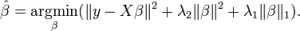A practical advantage of trading-off between Lasso and Ridge is that, it allows Elastic-Net to inherit some of Ridge’s stability under rotation.

#### Important Points:

• It encourages group effect in case of highly correlated variables
• There are no limitations on the number of selected variables
• It can suffer with double shrinkage

Beyond these 7 most commonly used regression techniques, you can also look at other models like Bayesian, Ecological and Robust regression.

## How to select the right regression model?

Life is usually simple, when you know only one or two techniques. One of the training institutes I know of tells their students – if the outcome is continuous – apply linear regression. If it is binary – use logistic regression! However, higher the number of options available at our disposal, more difficult it becomes to choose the right one. A similar case happens with regression models.

Within multiple types of regression models, it is important to choose the best suited technique based on type of independent and dependent variables, dimensionality in the data and other essential characteristics of the data. Below are the key factors that you should practice to select the right regression model:

1. Data exploration is an inevitable part of building predictive model. It should be you first step before selecting the right model like identify the relationship and impact of variables
2. To compare the goodness of fit for different models, we can analyse different metrics like statistical significance of parameters, R-square, Adjusted r-square, AIC, BIC and error term. Another one is the Mallow’s Cp criterion. This essentially checks for possible bias in your model, by comparing the model with all possible submodels (or a careful selection of them).
3. Cross-validation is the best way to evaluate models used for prediction. Here you divide your data set into two group (train and validate). A simple mean squared difference between the observed and predicted values give you a measure for the prediction accuracy.
4. If your data set has multiple confounding variables, you should not choose automatic model selection method because you do not want to put these in a model at the same time.
5. It’ll also depend on your objective. It can occur that a less powerful model is easy to implement as compared to a highly statistically significant model.
6. Regression regularization methods(Lasso, Ridge and ElasticNet) works well in case of high dimensionality and multicollinearity among the variables in the data set.

## End Note

By now, I hope you would have got an overview of regression. These regression techniques should be applied considering the conditions of data. One of the best trick to find out which technique to use, is by checking the family of variables i.e. discrete or continuous.

In this article, I discussed about 7 types of regression and some key facts associated with each technique. As somebody who’s new in this industry, I’d advise you to learn these techniques and later implement them in your models.

# Hypothesis testing steps

Question: What is the mean age of our population?

1. Data
• Take a random sample
• Sample mean = 27
• Sample std = 3
• n = 10
2. Assumption
• The population follows normal distribution
3. Hypothesis
• H0: Mean = 30
• Ha: Mean != 30
4. Pick test statistics
• Z = (SampleMean – M0)/sigma_sample = (SampleMean – M0)/(sigma/sqrt(n))
• Since we don’t know sigma, std of sample will be used instead of sigma –> No longer Z
5. Distribution
• t-distribution
6. Decision rule
• Minimize type 1 error (incorrect rejection of null hypothesis) = 5%
• ==> table look up to find t distribution critical values (standard error) (e.g. 2.262 for 5% error)
7. Calculate test statistic
• t = (SampleMean – M0) /(sigma/sqrt(n))
• t =(27-30)/(3/sqrt(10))= -3.15
8. Statistical decision
1. -3.15 < -2.262 ==> reject the null
2. confidence interval
3.9. Conclusion
10. P-value

# Hypothesis testing

What is p-value?

• The p-value is the probability of getting the observed value of the test statistic, or a value with even greater evidence against H0, if the null hypothesis is actually true.
• = A measure of the strength of the evidence against the null hypothesis
• The smaller the p-value, the greater the evidence against the null hypothesisRough guideline:

• p < 0.01: Very strong evidence against H0
• 0.01 < p < 0.05: Strong evidence against H0
• 0.05 < p < 0.10: Some weak  evidence against H0
• p > 0.10: Little or no evidence against Ho